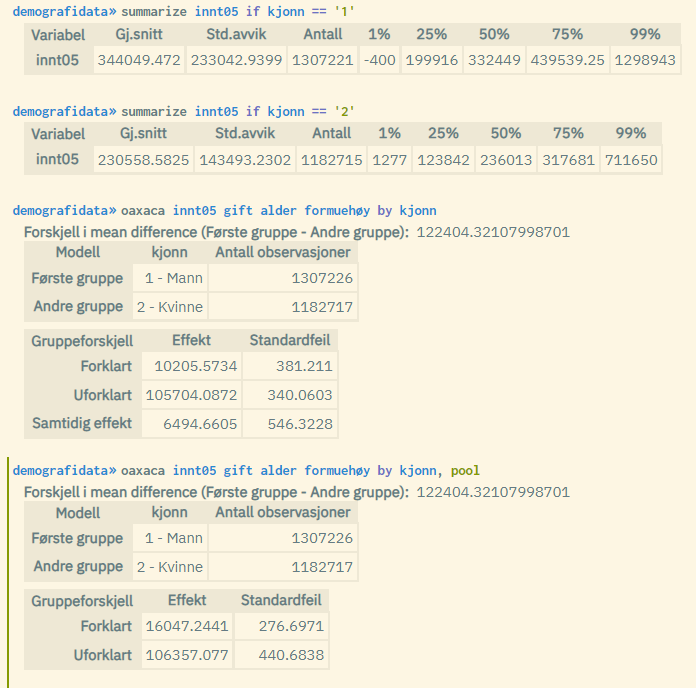# New regression functionality: Oaxaca

The analysis directory in microdata.no has been expanded with the new command `oaxaca`. This is a tool to measure whether there are systematic differences between two groups, e.g. men and women, and the differences are further decomposed into an explained and an unexplained component.

The `oaxaca` command performs a Blinder-Oaxaca decomposition that is used to explain differences in the mean value of the dependent variable for two groups. The difference is decomposed into two components: Explained difference (“between group”) and unexplained effect (coefficient effect). Similar to the `regress` command, continuous dependent variables such as e.g. wage are used. The difference is that you specify the two groups through the by-variable when using `oaxaca`.

The by-variable used for grouping must be categorical, but can have both numeric and alphanumeric value formats. The value that is ranked first (numerically or alphabetically) is linked to group 1. If the variable contains more than two values, the two values ​​that are ranked first are used, while the others are kept out of the analysis.

The standard solution used is “three-fold”, and you get the main numbers:

• The difference in the average value of the dependent variable measured for each of the two groups: mean(group1) – mean(group2)
• Decomposed difference: Explained, unexplained and simultaneous effect
• Number of units belonging to the two respective groups, as well as which value codes are used

By using the `pool` option, the system will use a so-called “two-fold pooled” approach where the decomposition uses the overall average as a reference value (simultaneous effect is not reported in this approach).

The most common use is to analyze systematic differences in economic variables such as wages, and compare men against women. But other types of groupings can also be used.

Example of using `oaxaca`:Note that the difference in mean value reported by oaxaca differs slightly from the difference found by using the summarize command on the dependent variable for each of the two groups. The reason is that descriptive statistics generated through commands like summarize are subject to winsorization (right and left censorship). Regression results from commands such as oaxaca, on the other hand, are not winsorized, and show the correct difference.